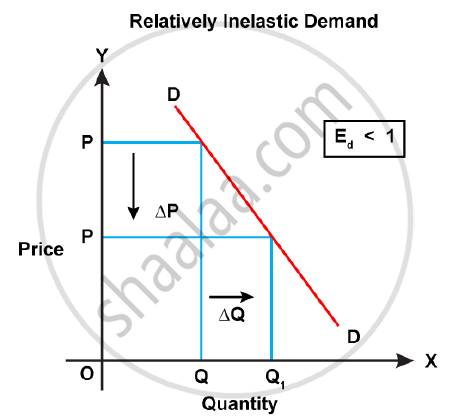Share

# With the Help of a Graph Explain the Relatively Inelastic Demand for a Commodity. - Economics

Course

#### Question

With the help of a graph explain the relatively inelastic demand for a commodity.

#### Solution

Relatively inelastic demand for a commodity:

When a large change in the price does not bring so much change in the demand, the demand is said to be inelastic. In this situation, the percentage change in demand is lesser than the percentage change in price. For example, a fall in the price of 10% leads to a rise in demand by only 5%. Such a situation will arise when the demand for a commodity is very urgent. Here, the numerical value of price elasticity of demand will be E < 1. The slope of the inelastic demand curve is steep.Is there an error in this question or solution?